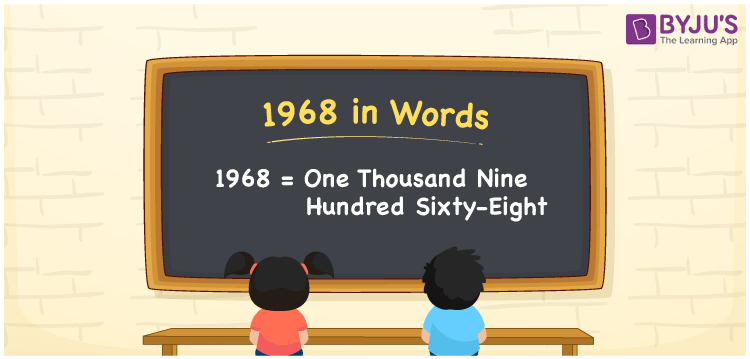# 1968 in Words

In English words, the number 1968 is written as “one thousand nine hundred sixty-eight”. In Mathematics, the number 1968 is said to be a cardinal number. For example. Akshaya was born in 1968. Also, it is a natural number and it exists between 1967 and 1969. Using the place values of 1968, we can easily write the number 1968 in words.

 1968 in Words: One Thousand Nine Hundred Sixty-eight. One Thousand Nine Hundred Sixty-eight in Numerical Form: 1968.

## 1968 in English Words## How to Write 1968 in Words?

Following is the place value table for the number 1968 up to four digits.

 Thousands Hundreds Tens Ones 1 9 6 8

The expanded form of 1968 is as follows:

= 1 × Thousand + 9 × Hundred + 6 × Ten + 8 × One

= 1 × 1000 + 9 × 100 + 6 × 10 + 8 × 1

= 1000 + 900 + 60 + 8

= 1968

= one thousand nine hundred sixty-eight

Hence, 1968 in words is one thousand nine hundred sixty-eight.

1968 in words – one thousand nine hundred sixty-eight

Is 1968 an odd number? – No

Is 1968 an even number? – Yes

Is 1968 a perfect square number? – No

Is 1968 a perfect cube number? – No

Is 1968 a prime number? – No

Is 1968 a composite number? – Yes

## Frequently Asked Questions on 1968 in Words

Q1

### Write 1968 in words.

1968 in words is one thousand nine hundred sixty-eight.

Q2

### Simplify 1900 + 68, and express it in words.

Simplifying 1900 + 68, we get 1968. Hence, 1968 in words is one thousand nine hundred sixty-eight.

Q3

### Is 1968 a composite number?

Yes, 1968 is a composite number.